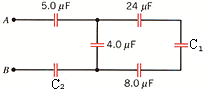# Problem: Determine the equivalent capacitance between A and B for the group of capacitors in the drawing. Let C1 = 13 µF and C2 = 6.0 µF.

###### FREE Expert Solution

Equivalent capacitance for parallel capacitors:

$\overline{){{\mathbf{C}}}_{{\mathbf{eq}}}{\mathbf{=}}{{\mathbf{C}}}_{{\mathbf{1}}}{\mathbf{+}}{{\mathbf{C}}}_{{\mathbf{2}}}}$

Equivalent capacitance for series capacitors:

$\overline{)\frac{\mathbf{1}}{{\mathbf{C}}_{\mathbf{eq}}}{\mathbf{=}}\frac{\mathbf{1}}{{\mathbf{C}}_{\mathbf{1}}}{\mathbf{+}}\frac{\mathbf{1}}{{\mathbf{C}}_{\mathbf{2}}}}$

The 24µF, C1, and 8.0 µF capacitors are in series:

95% (309 ratings)###### Problem DetailsDetermine the equivalent capacitance between A and B for the group of capacitors in the drawing. Let C1 = 13 µF and C2 = 6.0 µF.